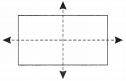## EX 13.2 QUESTION 1.

Find the number of lines of symmetry for each of the following shapes :Solution:(a)  4

(b)  4

(d) 1

(e) 6

(f)  6

(g) 0

(h) 0

(i)  3

## EX 13.2 QUESTION 2.

Copy the triangle in each of the following figures on squared paper. In each case, draw the line(s) of symmetry, if any and identify the type of triangle. (Some of you may like to trace the figures and try paper-folding first!)Solution:(a) It is an isosceles triangle having 1 symmetric line.

(b) This figure is an isosceles triangle having only 1 symmetric line.

(c) It is an isosceles right-angled triangle which has only 1 symmetric line.

(d) It is a scalene triangle. It has no symmetric line.

## EX 13.2 QUESTION 3.

Complete the following table.

 Shape Rough figure Number of lines of symmetry Equilateral triangle3 Square Rectangle Isosceles triangle Rhombus Circle

Solution:

 Shape Rough figure Number of lines of symmetry (a) Equilateral triangle3 (b) Square4 (c) Rectangle2 (d) Isosceles triangle1 (e) Rhombus2 (f) CircleInfinite

## EX 13.2 QUESTION 4.

Can you draw a triangle which has

(a) exactly one line of symmetry?

(b) exactly two lines of symmetry?

(c) exactly three lines of symmetry?

(d) no lines of symmetry?

Sketch a rough figure in each case.

Solution:## EX 13.2 QUESTION 5.

On a squared paper, sketch the following:

(a) A triangle with a horizontal line of symmetry but no vertical line of symmetry.

(b) A quadrilateral with both horizontal and vertical lines of symmetry.

(c) A quadrilateral with a horizontal line of symmetry but no vertical line of symmetry.

(d) A hexagon with exactly two lines of symmetry.

(e) A hexagon with six lines of symmetry.

Solution:## EX 13.2 QUESTION 6.

Trace each figure and draw the lines of symmetry, if any:Solution:(a) No line of symmetry.

(b) Two lines of symmetry.

(c) Four lines of symmetry.

(d) Two lines of symmetry.

(e) One horizontal line of symmetry.

(f) Two lines of symmetry.

## EX 13.2 QUESTION 7.

Consider the letters of English alphabets, A to Z. List among them the letters which have

(a) vertical lines of symmetry (like A)

(b) horizontal lines of symmetry (like B)

(c) no lines of symmetry (like Q)

Solution:

(a) Vertical lines = A, H, I, M, O, T, U, V, W, X, Y.

(b) Horizontal lines = B, C, D, E, H, I, K, O, X.

(c) No lines of symmetry = F, G, J, L, N, P, Q, R, S, Z.

## EX 13.2 QUESTION 8.

Given here are figures of a few folded sheets and designs drawn about the fold. In each case, draw a rough diagram of the complete figure that would be seen when the design is cut off.Solution:error: Content is protected !!